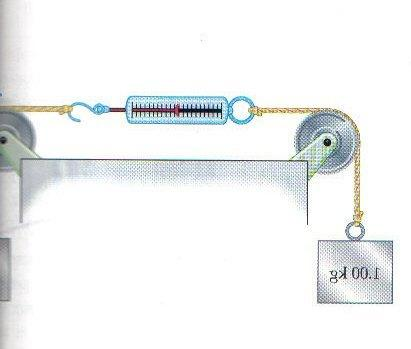# A pullmenhg

A pulley Problem

## Homework Statement

So, in the diagram attached one mass is 1 kg, and the other one is 2 kg.
and what would be reading on the scaleF=ma

## The Attempt at a Solution

I think this is very much like an elevator problem where the elevator is moving up, and we try to find the apparent weight, and here we have to find the apparent weight of 1 kg mass.
so, first I found overall a to be let's say a

Acceleration of the system = a
and then using that apparent weight formula <dunn want to derive it here>

Apparent weight = reading on the scale = 1 kg x (g+a)

#### Attachments

Last edited:

oops, and though the picture shows that the scale measuring hook is attached to 2 kg mass, however, since I made this question.. so, yea, actually the mass on the right should say 2 kg

Doc Al
Mentor
If I'm interpreting the problem correctly (the left side of the picture is cut off, so I'm not sure) then this has nothing to do with apparent weight on an accelerating elevator.

Here's a hint: The scale will display the tension in the rope. How would you solve for the tension? (Hint: Apply Newton's 2nd law to each mass.)

Last edited:
woaw!
thanks.
So, that would be

acceleration = (g(2-1) )/ total mass = g/3

and Net force on 1 kg = (g/3) [N]

and T = W+ (g/3)
=g+(g/3)

and so the reading would be = g (1+(1/3))

am i right?

I think i am using the right technique but math may be wrong..

Doc Al
Mentor
You are correct.

(But when presenting your work, always start from first principles--Newton's laws in this case--and show how you arrived at your answer. Don't just state your answer. I know how you got it, and you're correct, but if you were mistaken I'd have no idea where you went wrong.)